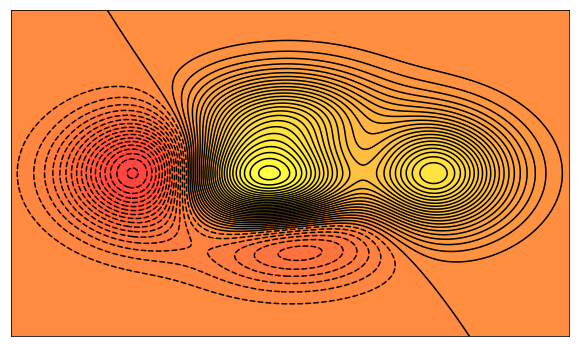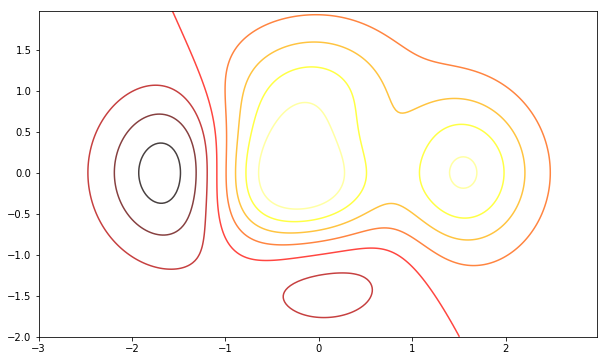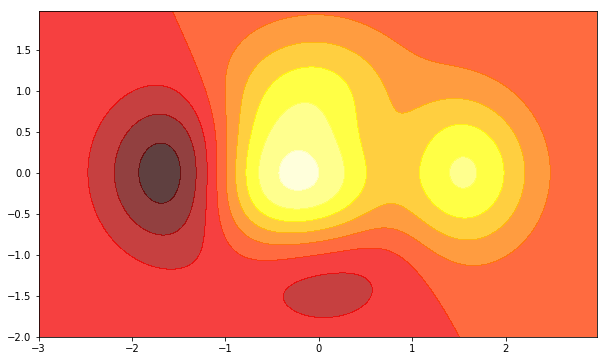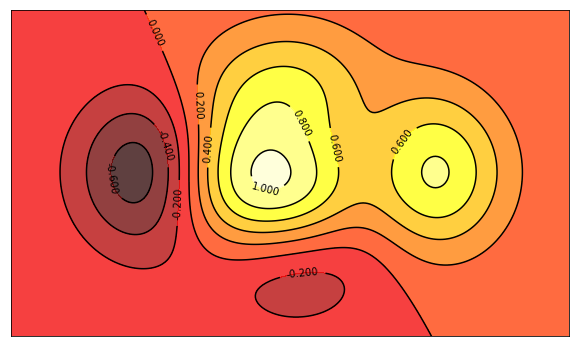# Matplotlib 中等高线图（contour）的绘制

Matplotlib

## 前言import matplotlib
import numpy as np
import matplotlib.cm as cm
import matplotlib.mlab as mlab
import matplotlib.pyplot as plt

%matplotlib inline

# 定义等高线高度函数
def f(x, y):
return (1 - x / 2 + x ** 5 + y ** 3) * np.exp(- x ** 2 - y ** 2)

# 数据数目
n = 256
# 定义x, y
x = np.linspace(-3, 3, n)
y = np.linspace(-3, 3, n)

# 生成网格数据
X, Y = np.meshgrid(x, y)


## 仅绘制等高线

# 设置图像大小尺寸
plt.figure(figsize=(10,6))

# 填充等高线的颜色, 8是等高线分为几部分
plt.contour(X, Y,f(X, Y), 8,alpha = 0.75, cmap = plt.cm.hot)## 仅填充等高区域颜色：

# 设置图像大小尺寸
plt.figure(figsize=(10,6))

# 填充等高区域的颜色, 8是等高线分为几部分
plt.contourf(X, Y,f(X, Y), 8, alpha = 0.75, cmap = plt.cm.hot)## 绘制完整的等高线图

# 设置图像大小尺寸
plt.figure(figsize=(10,6))

# 填充等高线的颜色, 8是等高线分为几部分
plt.contourf(X, Y, f(X, Y), 8, alpha = 0.75, cmap = plt.cm.hot)

C = plt.contour(X,Y, f(X,Y), 8, colors='black', linewidth=8)

# 绘制等高线数据
plt.clabel(C, inline = True, fontsize = 10)

# 去除坐标轴
plt.xticks(())
plt.yticks(())

plt.show()